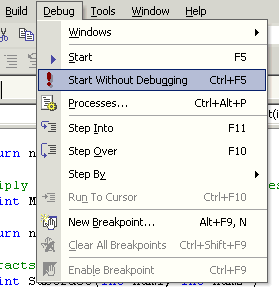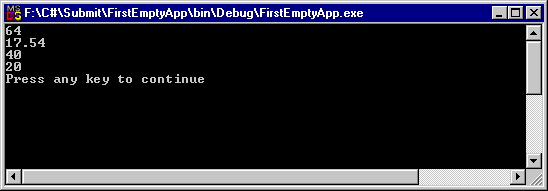# Creating an Empty Project using VS.NET

There are many occasions when you need to create an empty project and add classes and methods late to the project. There are many tutorial, which will show you how to create Windows or Web applications, but you rarely see a tutorial building an empty project.

This tutorial guides you towards your first C# Empty project step by step. After that I'll show you how to add a class to the project and few members to the class and call this class members from the main method.

Step 1: Create an Empty Project

Select File->New->Project->Visual C# Projects->Empty Project. Select your project name and appropriate directory using Browse button. See Figure 1.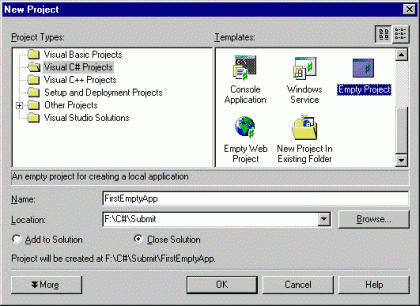Figure 1.

When you create an empty project, there is no class available in the project.

Step 2: Adding an Empty Class

Right click on the project in Solution Explorer and click Add->Add Class. See Figure 2.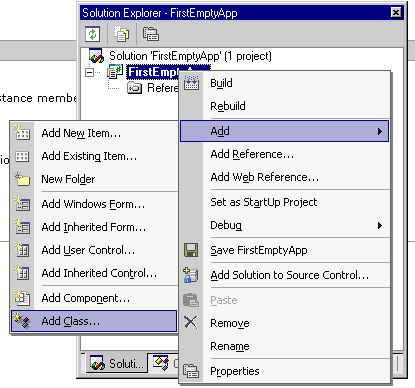Figure 2.

Add Class option launches Add New Item dialog, where you can select from many items. I select Class option as you can see from Figure 3. Write name of your class and click Open. I put my class name as mcbClass. Click Open here.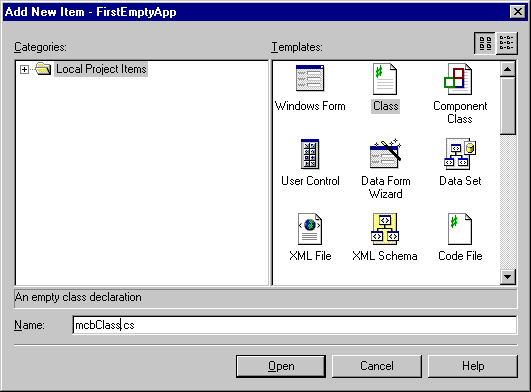Now you have added a class to your project. Visual Studio create a skeleton of the class for you. It looks like here:

using System;
|
namespace
FirstEmptyApp
{
///
<summary>
///
Summary description for mcbClass.
/// </summary>

public class
mcbClass
{
public
mcbClass()
{
//
// TODO: Add constructor logic here
//
}
}
}

Now it time to add functionality to your class. I add few methods to this class. As you can see from the following listing, I add Add, Multiply, Subtract, and Sqaure methods to the class.

public class mcbClass
{
public
mcbClass()
{
//
// TODO: Add constructor logic here
//
}
// Square function
public int Square(int
num)
{
return
num*num;
}
// Add two integers and returns the sum
num2 )
{
return
num1 + num2;
}
// Add two integers and returns the sum
num2 )
{
return
num1 + num2;
}
// Multiply two integers and retuns the result
public int Multiply(int num1, int
num2 )
{
return
num1 * num2;
}
// Subtracts small number from big number
public int Subtract(int num1, int
num2 )
{
if
( num1 > num2 )
{
return
num1 - num2;
}
return
num2 - num1;
}
}

Now your class is ready. Next step is to add a Main method to the project. The Main method executes when you start an application, means it's the entry point of an application. See BOLD part in the following listing.

I add Main method to the same namespace just after mcbClass. Now the entire code looks like following listing.

using System;
namespace
FirstEmptyApp
{
/// <summary>

///
Summary description for mcbClass.
/// </summary>

public class
mcbClass
{
public
mcbClass()
{
//
// TODO: Add constructor logic here
//
}
// Square function
public int Square(int
num)
{
return
num*num;
}
// Add two integers and returns the sum
num2 )
{
return
num1 + num2;
}
// Add two integers and returns the sum
num2 )
{
return
num1 + num2;
}
// Multiply two integers and retuns the result
public int Multiply(int num1, int
num2 )
{
return
num1 * num2;
}
// Subtracts small number from big number
public int Subtract(int num1, int
num2 )
{
if
( num1 > num2 )
{
return
num1 - num2;
}
return
num2 - num1;
}
}
// Call mcClass from Main
public class
Class1
{
static void
Main()
{
mcbClass sq =
new
mcbClass();
Console.WriteLine( sq.Square(8).ToString());
Console.WriteLine( sq.Multiply(5,8).ToString());
Console.WriteLine( sq.Subtract(22, 42).ToString());
}
}

Step 4: Build and Run the Project